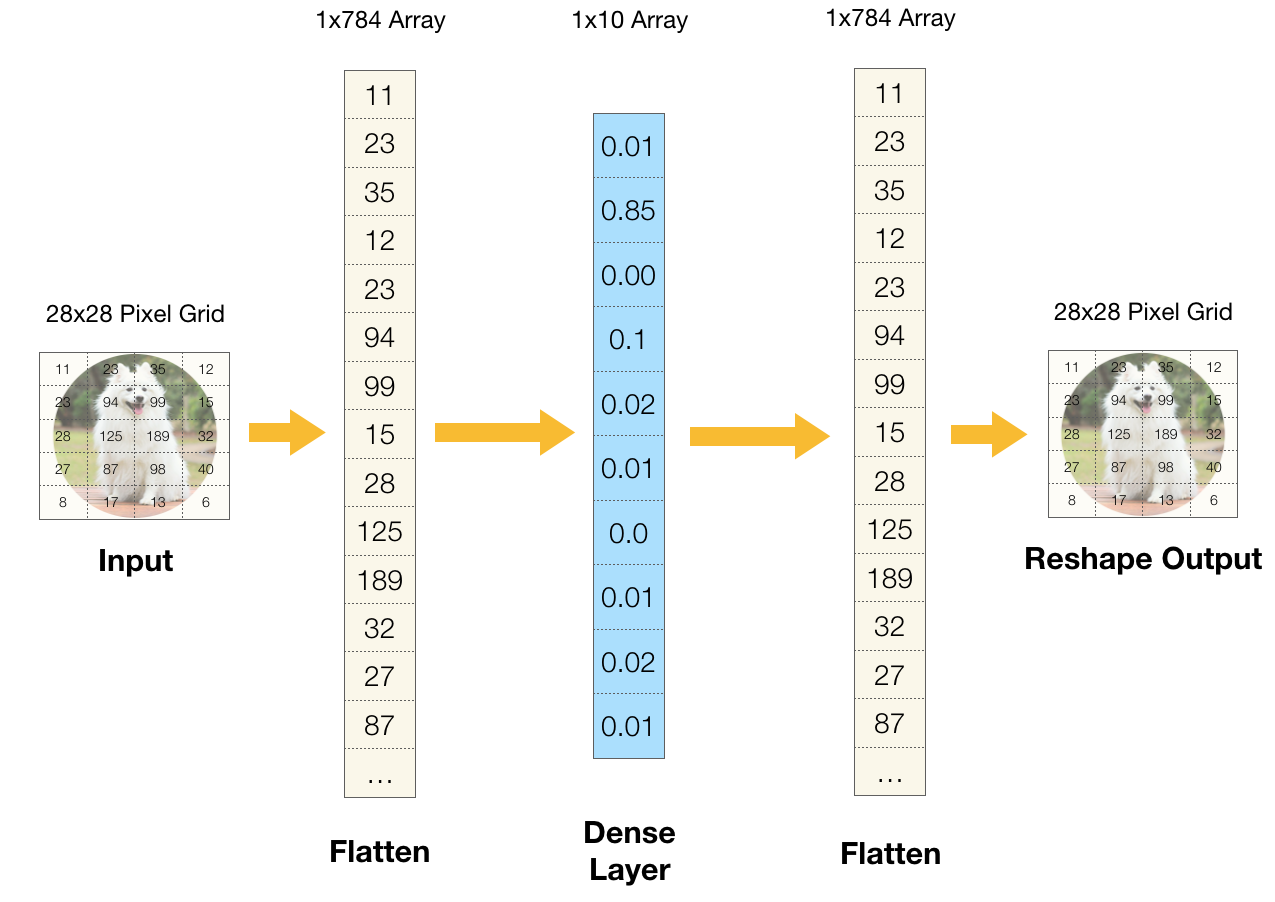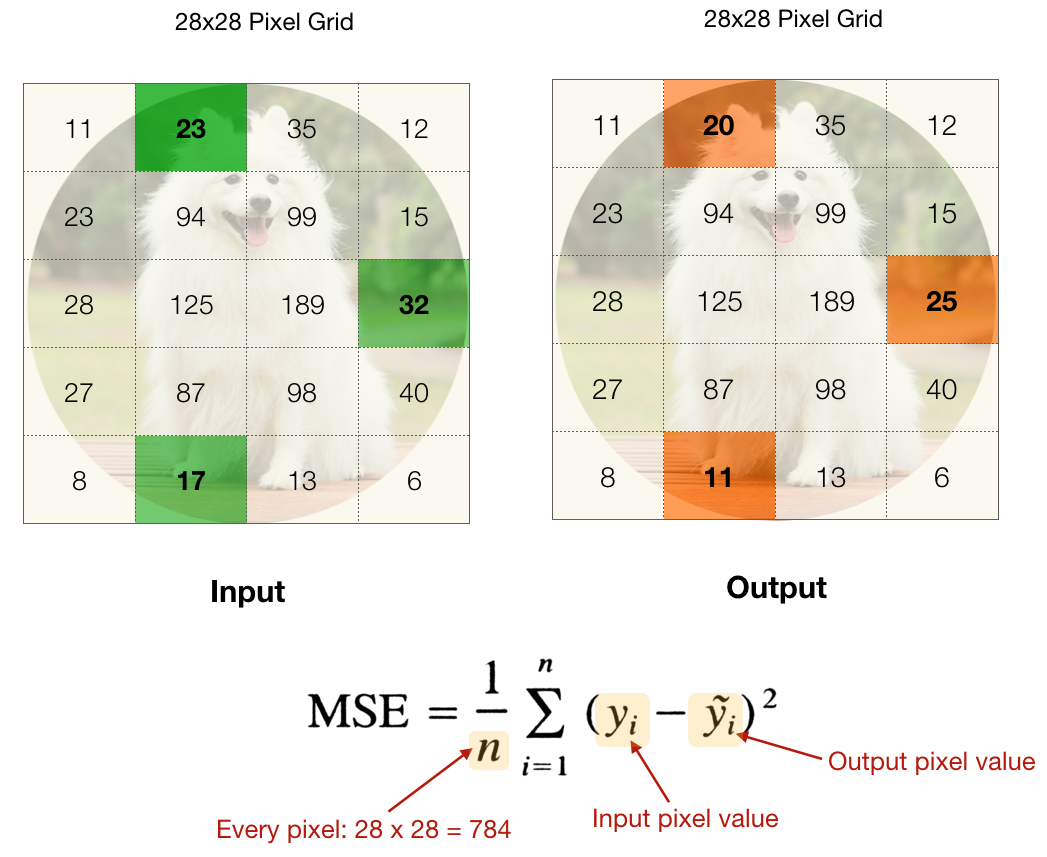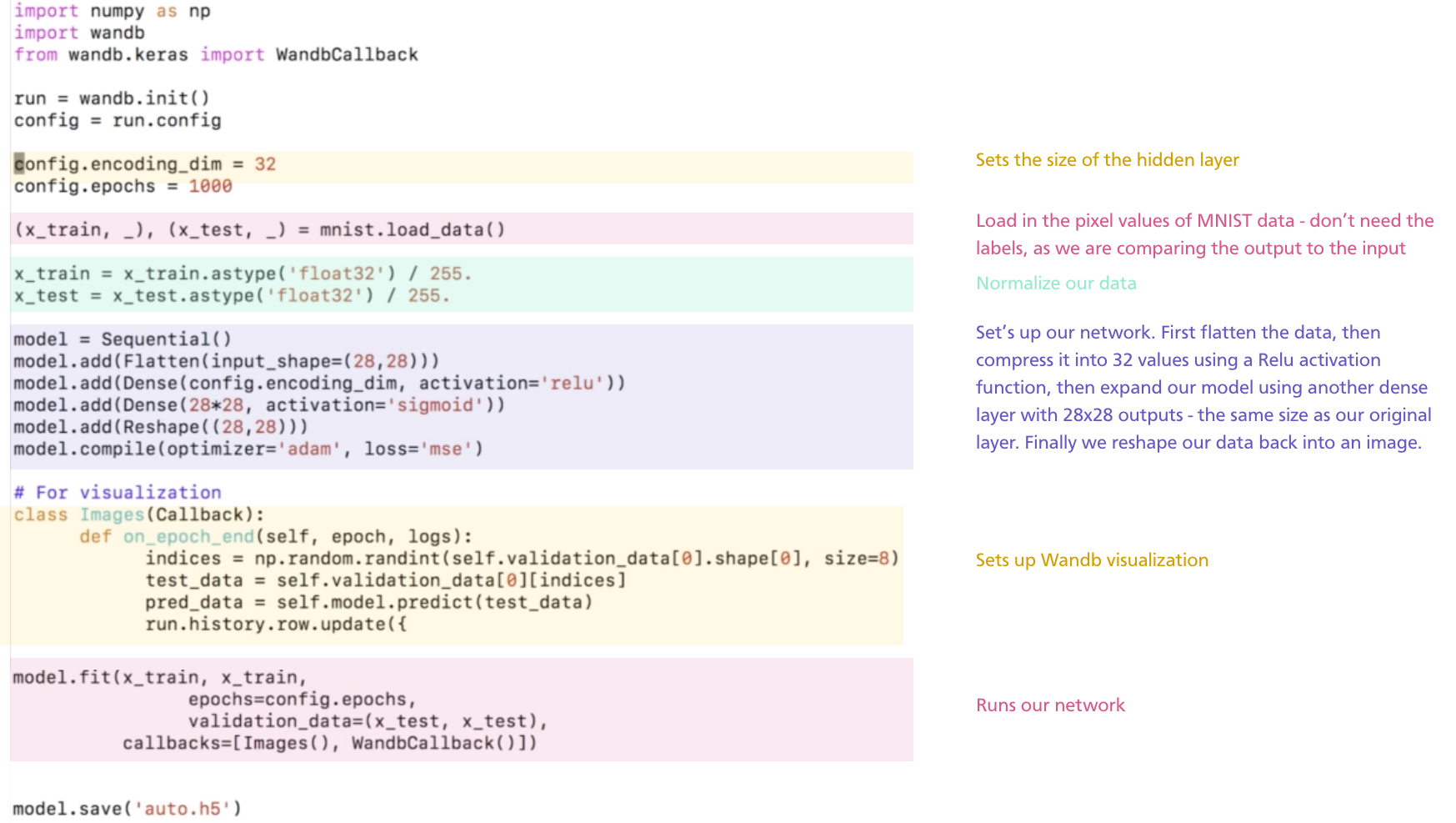# 4. Autoencoders

Autoencoders are a really cool application of neural nets, and a great place to start if you want to learn more about generative adversarial networks (GANs).  Autoencoders are basically perceptrons with a twist. The input and the output of an autoencoder are the same. We can use autoencoders to compress information, generate synthetic images and remove noise from images.

What is the intuition for an autoencoder? Imagine you see a picture of someone, and have to describe it to a friend in 16 words, and then they have to draw that person. You will have to really distill the characteristics of that person to only fit in 16 words. Similarly, a neural network is going to do the same thing to another neural network, using only the hidden layer to describe the input. This is really useful for compression.

Let’s walk through how the autoencoder works. The code for this is in autoencoders/autoencoders.py. The model takes in an image, flattens it and runs a dense array of perceptrons on it. It is then going to run another array of perceptrons with outputs equal to the size of the original image.  Finally, we reshape the output into the form of the original image.# Loss functions for Autoencoders

For autoencoders, the loss is the difference between pixels in the input image and the output image. We can use a loss function to calculate the overall loss of the model - in this case the Mean Square Error loss function makes sense, but you can definitely try others. We then feed this loss value into a gradient descent function to optimize our weights.# Hidden Layers

The size of the middle hidden layer affects the compression of the image. If the hidden layer is large, the network should be able to easily recreate the input image. However, if the hidden layer is small, then the network will have to use it as efficiently as possible. This technique is used in compression: gradient descent will force the network to compress the pixel values into a smaller space.  We could save each image with the layers that reduce the image down to the middle layers and then decompress the image with the layers that expand the image from the middle layer.

# Code

Most Let's see how this all comes together and walk through the code in autoencoders.py.Run this code to see how our original images compare to the output images.

# Applying a CNN to the Autoencoder

Open up autoencoder_cnn.py.  This is a lot like autoencoder.py but the architecture is now convolutional.  Just like in the previous tutorial, we need to reshape the data to 28 by 28 by 1 to work with the Conv2d layers.  In the convolutional layer, we use a special padding=same so that the input and output shape of the convolution is the same. Then we perform max pooling to reduce the size. We add another convolutional layer and then an upsampling layer which just repeats rows and columns to make the image twice as big.  Finally, we add one more convolutional layer with a single output to match the shape of the input.

This network takes a long time to train but it works really well with a smaller number of parameters. As a challenge, you may want to try to do the same with the denoising net.